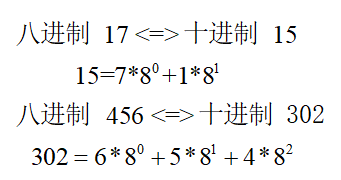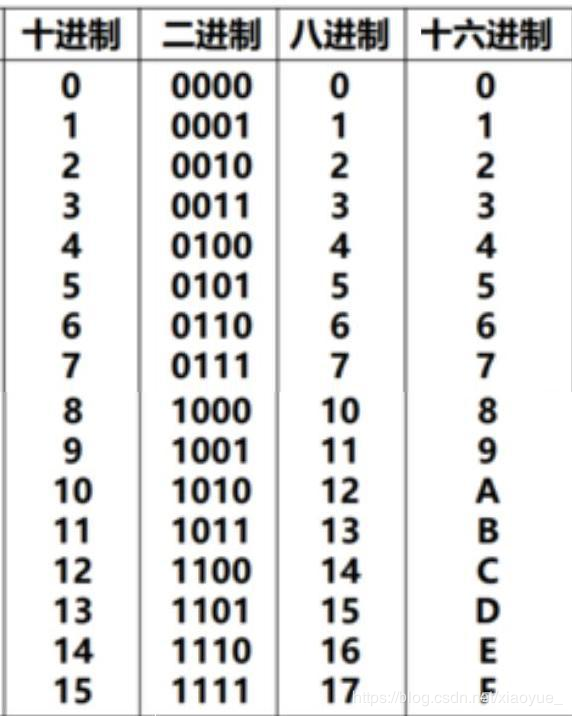• 十进制转换为二进制 题目 输入一个正整数n，将其转换为二进制后输出。要求定义并调用函数dectobin(n)，它的功能是输出n的二进制数。 例如，调用dectobin(10)，输出1010。 输入 输入一个10进制整数。 输出 输出其二...
十进制转换为二进制
题目 输入一个正整数n，将其转换为二进制后输出。要求定义并调用函数dectobin(n)，它的功能是输出n的二进制数。
例如，调用dectobin(10)，输出1010。
输入
输入一个10进制整数。
输出
输出其二进制的形式。
样例输入 Copy
100
样例输出	Copy
1100100*/
为什么这样写不对？
#include<stdio.h>
int dectobin(int n);
int main(void)
{
int n;
scanf("%d",&n);
dectobin(n);
return 0;

}
int dectobin(int n)
{
int i,j,t,count=0;
int a;
while(n!=0)
{
for(i=0;a[i]!=’\0’;i++)
{
t=n%2;
n=n/2;
a[i]=t;
count++;
}
}
for(j=count-1;j>=0;j–)
printf("%d",a[j]);
return 0;
}


展开全文c语言
• 我正在尝试使用Java将用户输入的十进制转换为二进制数。我收到错误package reversedBinary;import java.util.Scanner;public class ReversedBinary {public static void main(String[] args) {int number;Scanner in...
我正在尝试使用Java将用户输入的十进制转换为二进制数。我收到错误package reversedBinary;import java.util.Scanner;public class ReversedBinary {public static void main(String[] args) {int number;Scanner in = new Scanner(System.in);System.out.println("Enter a positive integer");number=in.nextInt();if (number <0)System.out.println("Error: Not a positive integer");else {System.out.print("Convert to binary is:");System.out.print(binaryform(number));}}private static Object binaryform(int number) {int remainder;if (number <=1) {System.out.print(number);}remainder= number %2;binaryform(number >>1);System.out.print(remainder);{return null;} } }在Java中如何将十进制转换为二进制？
展开全文• //题目：八进制转换为十进制 #include<stdio.h> #include<math.h> int main() { int i=0,n,tmp,sum=0; scanf("%d",&n); while(n) { tmp=n%10; n=n/10; sum+=tmp*pow(8,i); i++;...
基本思想:   八进制转十进制，二进制转十进制都一样的道理八进制 --> 十进制
//【程序48】
//题目：八进制-->十进制
#include<stdio.h>
#include<math.h>
int main()
{
int i=0,n,tmp,sum=0;
scanf("%d",&n);
while(n)
{
tmp=n%10;
n=n/10;
sum+=tmp*pow(8,i);
i++;
}
printf("%d",sum);
return 0;
}

二进制 --> 十进制
#include<stdio.h> //二进制-->十进制
#include<math.h>
int main()
{
int i=0,n,tmp,sum=0;
scanf("%d",&n);
while(n)
{
tmp=n%10;
n=n/10;
sum+=tmp*pow(2,i);
i++;
}
printf("%d",sum);
return 0;
}

十进制 --> 二进制
#include<stdio.h>  //十进制-->二进制
int main()
{
int i=0,n,str;
scanf("%d",&n);
while(n)
{
str[i]=n%2;
n=n/2;
i++;
}
for(int j=i-1;j>=0;j--)
printf("%d",str[j]);
return 0;
}

十进制 --> 八进制
#include<stdio.h>  //十进制-->八进制
int main()
{
int i=0,n,str;
scanf("%d",&n);
while(n)
{
str[i]=n%8;
n=n/8;
i++;
}
for(int j=i-1;j>=0;j--)
printf("%d",str[j]);
return 0;
}

十六进制 --> 十进制
#include<stdio.h> //十六进制-->十进制 eg. (十六进制)AB-->171(十进制)
#include<math.h>                                  //ABCD-->43981
int main()
{
int i,j,k=0;
long int sum=0,tmp;
char str,a;
gets(str);
for(i=0;str[i]!='\0';i++)
{
if(str[i]>='0'&&str[i]<='9')
a[i]=str[i]-'0';
if(str[i]>='a'&&str[i]<='z')
a[i]=str[i]-'a'+1+9;
if(str[i]>='A'&&str[i]<='Z')
a[i]=str[i]-'A'+1+9;
}
for(j=i-1;j>=0;j--)
{
tmp=a[j]*pow(16,k);
sum+=tmp;
k++;
}
printf("%ld",sum);
return 0;
}
//#include<stdio.h>         //方法二：参看大佬的解法
//int main()                //16-->10进制
//{
//	int num = 0;
//    printf("输入一个十六进制数: ");
//    scanf("%x", &num);
//    printf("十六进制数 %x 转换为十进制为 %d\n", num, num);
//	return 0;
//}

十进制 --> 十六进制
#include<stdio.h>
int main()
{
int n,i=0,j;
char ten,str="0123456789ABCDEF";  //10进制-->16进制
scanf("%d",&n);                           //46-->2E; 59-->3B
while(n)                                  //46= 2*16^1 + 14*16^0
{
ten[i++]=str[n%16];
n=n/16;
}
for(j=i-1;j>=0;j--)
printf("%c",ten[j]);
return 0;
}展开全文c语言
• 听说优酷前端面试有问进制数转换的，甚至是负正整数转换为二进制，那就索性一次整个明白~ 　原文来自：http://jingyan.baidu.com/article/597a0643614568312b5243c0.html 十进制转成二进制主要有以下3种： 　正...
　　听说优酷前端面试有问进制数转换的，甚至是负正整数转换为二进制，那就索性一次整个明白~
原文来自：http://jingyan.baidu.com/article/597a0643614568312b5243c0.html
十进制转成二进制主要有以下3种：
正整数转二进制、负整数转二进制、小数转二进制
1、  正整数转成二进制。
口诀：除二取余，倒序排列，高位补零。
举个栗子：42转化为二进制（本来打算自己画张图的，太麻烦，直接粘百度到的图吧~）42 除以2得到的余数（右边）为010101，然后倒着排一下就是取到的二进制了（如下图）计算机内部表示数的字节单位是定长的，如8位，16位，或32位。所以，位数不够时，高位补零，所说，如图3所示，42转换成二进制以后就是。00101010，也即规范的写法为（42）10=（00101010）22、  负正整数转成二进制。
先是将对应的正整数转换成二进制后，对二进制取反，然后对结果再加一。还以42为例，负整数就是-42，如图4所示为方法解释。最后即为：（-42）10=（11010110）2.2、  小数转成二进制。
对小数点以后的数乘以2，有一个结果吧，取结果的整数部分（不是1就是0喽），然后再用小数部分再乘以2，再取结果的整数部分……以此类推，直到小数部分为0或者位数已经够了就OK了。然后把取的整数部分按先后次序排列就OK了，就构成了二进制小数部分的序列，举个例子吧，比如0.125，如图5所示。如果小数的整数部分有大于0的整数时该如何转换呢？如以上整数转换成二进制，小数转换成二进制，然后加在一起就OK了，如图6所示。二进制转成十进制：

首先将二进制数补齐位数，首位如果是0就代表是正整数，如果首位是1则代表是负整数。
先看首位是0的正整数，补齐位数以后，将二进制中的位数分别将下边对应的值相乘，然后相加得到的就为十进制，比如1010转换为十进制，方法如图7所示。若二进制补足位数后首位为1时，就需要先取反再换算：例如，11101011，首位为1，那么就先取反吧：-00010100，然后算一下10100对应的十进制为20，所以对应的十进制为-20，方法如图8所示。有小数的二进制转换为十进制：例如0.1101转换为十进制的方法：将二进制中的四位数分别于下边（如图9所示）对应的值相乘后相加得到的值即为换算后的十进制。转载于:https://www.cnblogs.com/zhangym118/p/5949997.html
展开全文• 十进制转换为十六进制，也就是 采用 除k取余法 ，直接让10处以16，再对 数据如果小于10和大于10进行处理，代码如下： #include<iostream> #include<string> using namespace std; int main() { ...C++ 16进制
• 用Python程序将十进制转换为二进制十进制转换成二进制通过将数字连续除以2并以相反顺序打印其余部分，将十进制数转换为二进制。在下面的程序中，我们将学习使用递归函数将十进制数转换为二进制数，代码如下：def ...
• 介绍了C++ 十进制转换为二进制的实例代码，有需要的朋友可以参考一下
• 十进制转换为二进制代码 十进制转换为二进制： 十进制如何转二进制：将该数字不断除以2直到商为零，然后将余数由下至上依次写出，即可得到该数字的二进制表示。 以将数字21转化为二进制为例 当商为零时，将余数由下...算法 c++
• 1.十进制转换为二进制 #include using namespace std; int main(){  int N;  cout  int aa;  cin>>N;  int temp;  temp = N;  int num;  int i=0;  while(temp !=0)  {c++ integer input
• labview 十进制转换为二进制,比较简单的一种方法，还可以用在其他方面labview
• C语言-顺序栈实现十进制转换为二进制-八进制-十六进制
• 主要介绍了C语言用栈实现十进制转换为二进制的方法,结合实例形式分析了C语言栈的定义及进制转换使用技巧,需要的朋友可以参考下
• 十进制转换为二进制和十六进制将十进制转换为二进制和十六进制
• 十进制转换为二进制和十六进制 一.十进制数转化为二进制 整数除以2，商继续除以2，得到0为止。 以23为例： 23/2 11余1 11/2 5 余 1 5 /2 2余 1 2 /2 1余 0 1 /2 0余 1 对于其余数从上到下为二进制的低位到高位;...
• 用Python程序将十进制转换为二进制十进制转换成二进制通过将数字连续除以2并以相反顺序打印其余部分，将十进制数转换为二进制。在下面的程序中，我们将学习使用递归函数将十进制数转换为二进制数，代码如下：def ...
• 位运算在计算机编程中经常...比如下图中的例子，十进制的13转为二进制表示，则1101。十进制二进制二进制十进制的原理：二进制数的每一位乘以2的n次方，n 从零开始，每次加一，最后累加。下面是将二进制的1101...
• 十进制转换为二进制，可参考二、八、十、十六进制转换 分析： 将一串未知数字按照某种指定顺序输出时，可以考虑先将每个数字分别存入数组中，通过数组下标来确定位置再输出 注意： int型占16个二进制位 二进制数...
• 十进制转换为二进制 #!/usr/bin/python3 def mybin(a):#自定义函数去除系统自带的多余0b a=bin(a) a=a.replace('0b','') print(a) mybin(17) #结果 10001 十进制转换为二进制 #!/usr/bin/python3 ...
• toBinaryString(int i) //返回int变量的二进制表示的字符串 toHexString(int i) //返回int变量的十六进制表示的字符串 ...//N进制转换十进制，返回类型int,第一个参数字符串，第二个参数进制 具体
• 1 //利用栈实现十进制转换为二进制2 package classwork9;34 import java.util.Scanner;5 import java.util.Stack;67 public class Jinzhizhuanhuan {8 public static int zhuanhuan(int x) {9 Stack a = new Stack()......